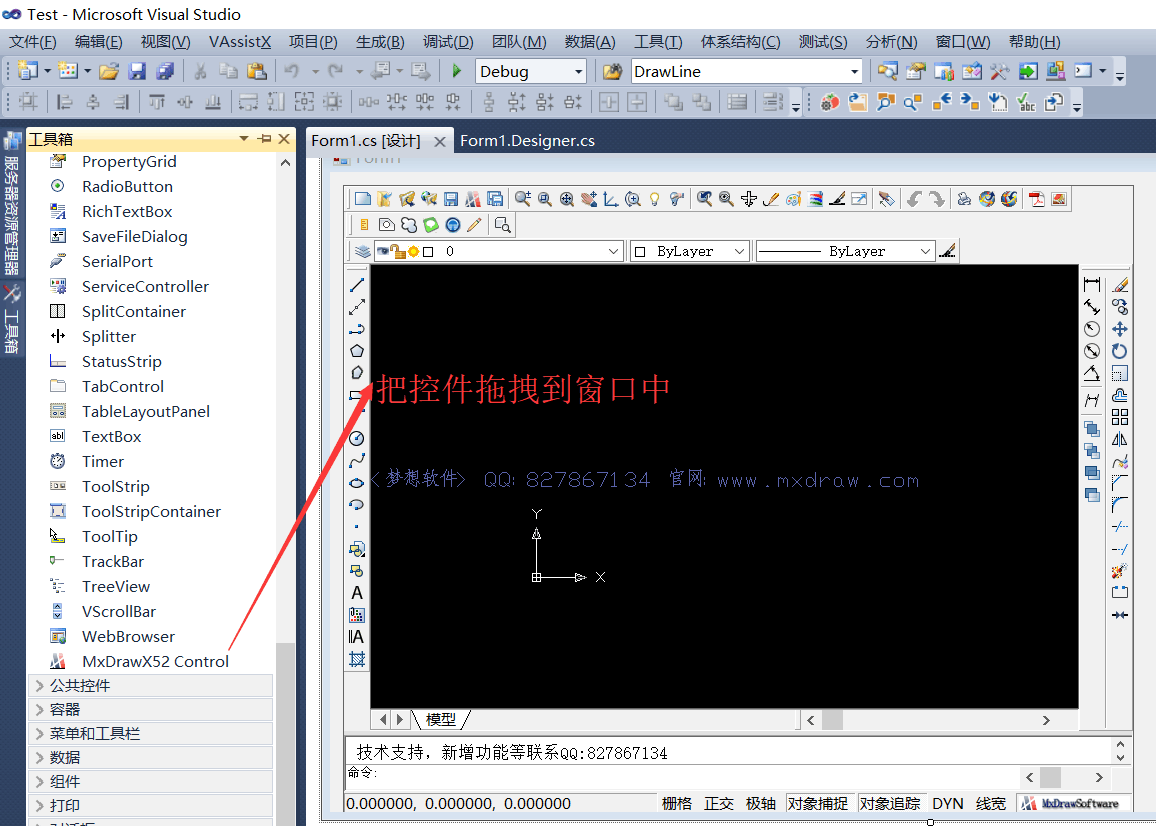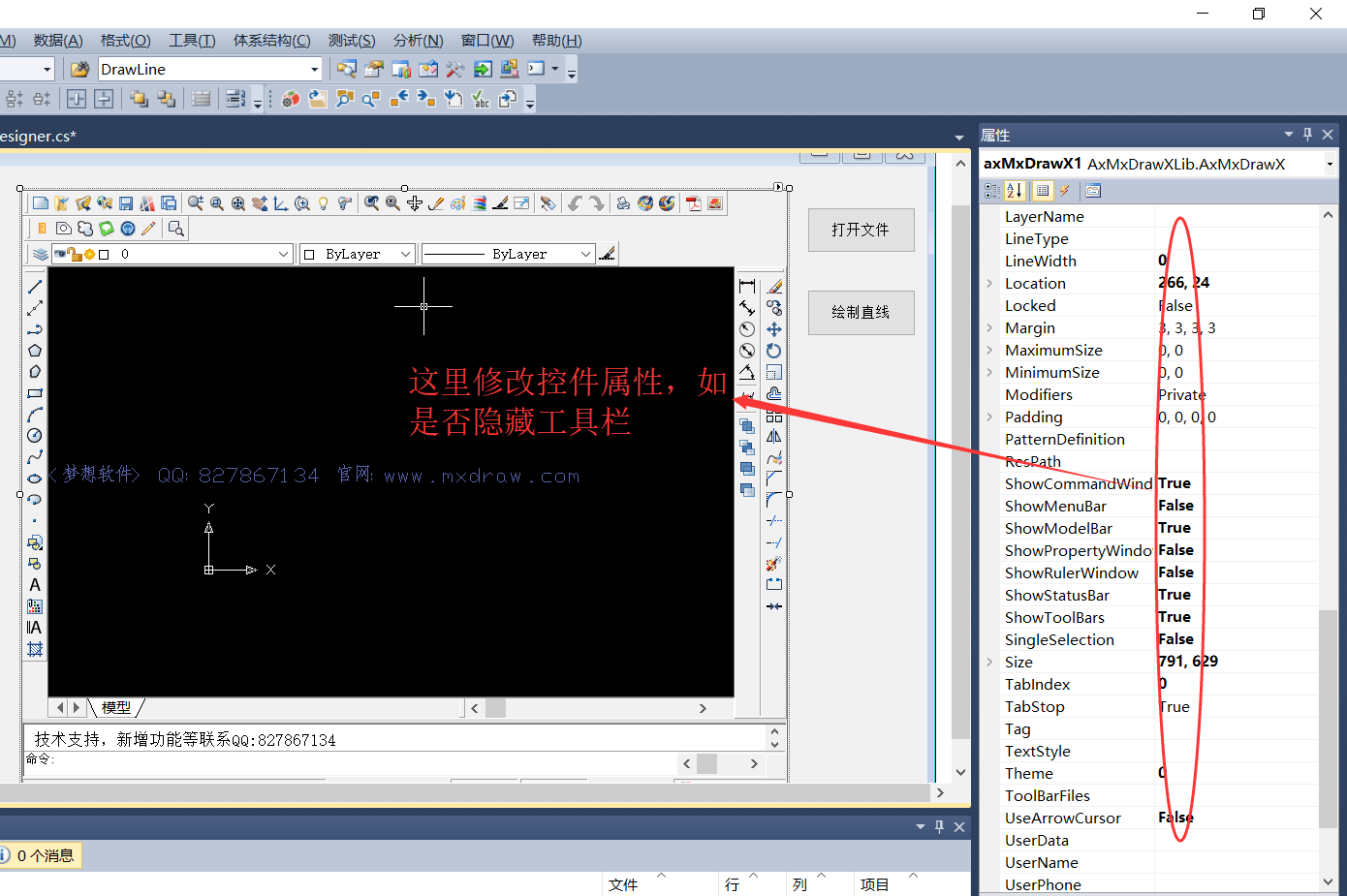.`using MxDrawXLib;`

```//打开文件对话框
OpenFileDialog ofd = new OpenFileDialog();
ofd.Filter = "dwg 文件(*.dwg)|*.dwg";

if(ofd.ShowDialog() ==  DialogResult.OK)
{
//打开文件
axMxDrawX1.OpenDwgFile(ofd.FileName);
}```

```//实例化控件工具集合
MxDrawUtility mxUtility = new MxDrawUtility();
//点取第一点
MxDrawPoint point = (MxDrawPoint)(mxUtility.GetPoint(null, "点取第一点:"));

if (point == null)
{
MessageBox.Show("用户取消...");
return;
}
//点取第二点
MxDrawPoint point2 = (MxDrawPoint)(mxUtility.GetPoint(point, "点取第二点:"));

if (point2 == null)
{
MessageBox.Show("用户取消...");
return;
}
//调用绘制直线函数
axMxDrawX1.DrawLine(point.x, point.y, point2.x, point2.y);```